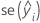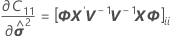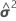# Methods and formulas for conditional fits and residuals in Fit Mixed Effects Model

Select the method or formula of your choice.

## Conditional fitted value

The conditional fitted values are calculated using the following equation:

For further details on the notation, see the Methods section, the Test of Fixed Effects Section, and the Random Effect Predictions section.

## Standard error of the conditional fitted value (SE Fit)

The standard errors of the conditional fitted values are the square roots of the diagonal elements of the following matrix:

where

## Confidence intervals for conditional means

The range in which the mean response for a given set of predictor values is expected to fall.is the conditional fit.is the standard error of he fit.

The degrees of freedom use this formula for the conditional case:

where
and### Notation

TermDescription
Wthe asymptotic variance-covariance matrix ofFor further details on the notation, go to the Methods section and the Test of Fixed Effects section.

## Conditional residuals

A residual is the difference between an observed value and a fitted value. This part of the observation is not explained by the fitted model.

Theconditional residual is calculated by the following expression:

### Notation

TermDescription
yitheresponse valuetheconditional fitted value

## Standardized conditional residuals

Standardized residuals are also called "internally Studentized residuals."

where the standard deviation of the residual is the appropriate diagonal square root of the residual variance matrix:

where

### Notation

TermDescription
eitheresidual
Std(ei)the standard deviation of theresidual
By using this site you agree to the use of cookies for analytics and personalized content.  Read our policy